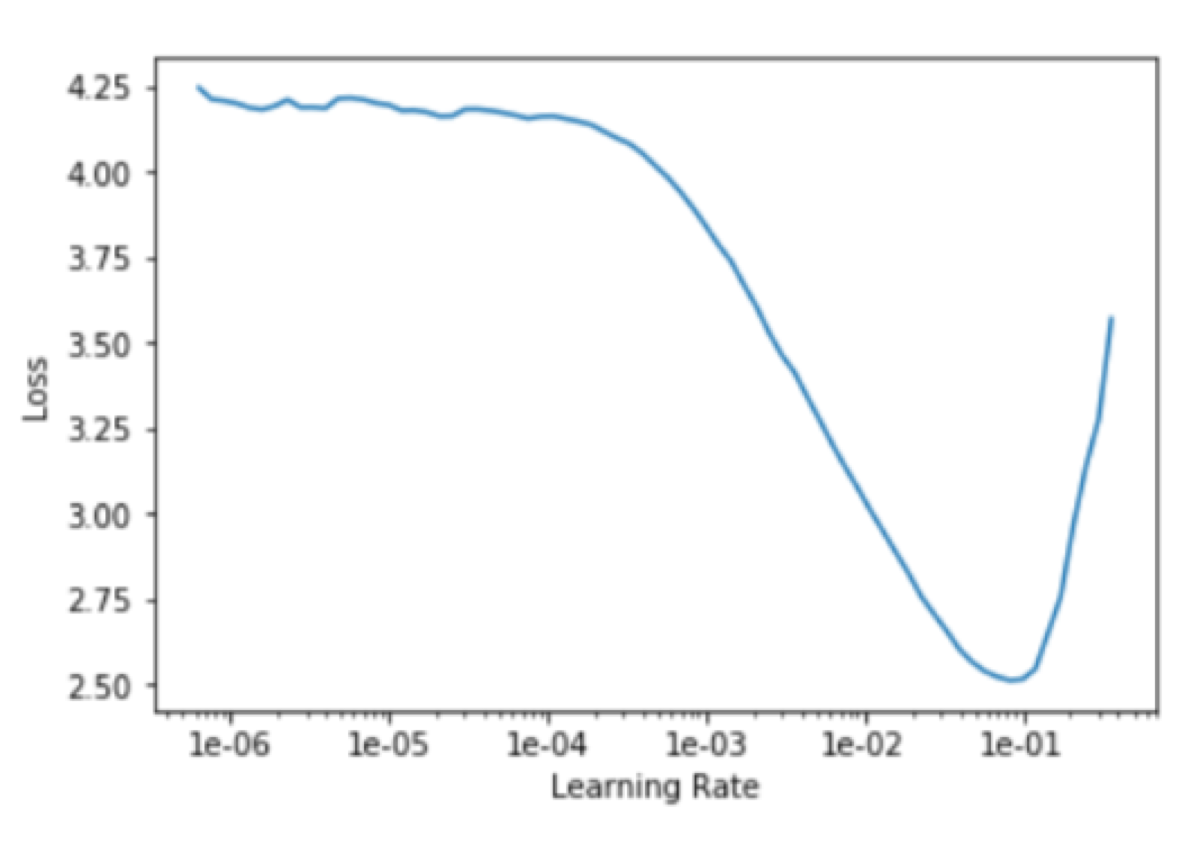I used a pre-learned resnet50 network. I had just finished transfer learning (training only the last layer of a pre-learned neural network on a new dataset) and the only thing I needed to do was fine-tuning the network so it could fit better to the categories of my dataset.

Fortunately, I decided to use fast.ai, so the task was relatively easy. Just set the learning rates, give the network new examples and let it learn. It turned out that the learning rate was a little bit problematic.

``````learn.unfreeze()
learn.fit_one_cycle(number_of_epochs, max_lr=[first_layers, middle_layers, last_layers])
``````

Usually, when we train a neural network, we determine the optimal learning rate using the method described in Leslie N. Smith’s paper: “Cyclical Learning Rates for Training Neural Networks.”

That was precisely what I have done. I used the lr_find function available in fast.ai to get the chart of loss values and their corresponding learning rates.Remember that 1e-02 = 1 * 10^(-2) and 1e-01 = 1 * 10^(-1)

The optimal learning rate is supposed to be the value that gives us the fastest decrease in loss. It seemed that something between 1e-2 and 1e-1 would do the job.

To be sure I plotted a chart of loss derivatives with respect to the learning rate using Pavel Surmenok’s code.

Now, I was sure. The optimal value was right in between of 1e-2 and 1e-1, so I set the learning rate of the last layers to 0.055. For the first and middle layers, I set 1e-5 and 1e-4 respectively, because I did not want to change them a lot. It was wrong. The results were terrible. In every epoch of the training, both training loss and validation loss were getting worse and worse.

## What have I forgotten?

That is the method of finding the optimal learning rate for training a new network. The task I was doing was just fine-tuning. I should not use the value that change the loss a lot. My optimal values were the ones that make only small differences. Let’s look at the derivatives chart again. What makes the smallest difference? The loss values between 1e-6 and 1e-4!

## Why?

Remember that I used a pre-learned model, trained it on my dataset and them just had to unfreeze all layers and do some fine tuning. At this stage, it is too late to make massive changes. It is the last tweak. Like adding salt to a dish while cooking. Just like the “Salt bae” ;)

Older post

## F1 score explained

The mathematics behind F1 score.